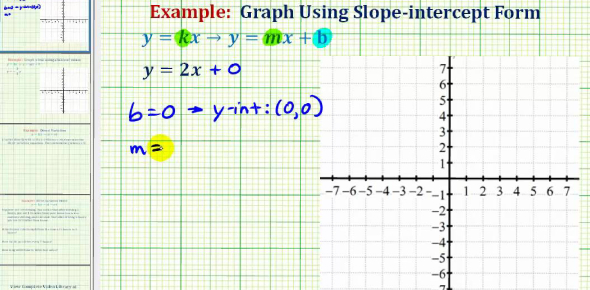# Alg. 1: Direct Variation

5 Questions | Total Attempts: 1539SettingsAlgebra 1, Direct Variation quiz. If x=a and y=b find y/x if x/y=cWord ProblemsWrite EquationsFormula: y=kx

• 1.
The amount of money raised at a charity fundraiser is directly proportional to the number of attendees.  The amount of money raised for five attendees was \$100.  How much money will  be raised for 60 attendees?Hint: \$x,xxx
• 2.
Write an equation for the direct variation. Do not solve.If x=3 when y=4, find y when x=24Hint: y=kx
• 3.
Write an equation for the direct variation. Do not solve.If y = 20 when x = 4, find y when x = 9Hint: y=kx
• 4.
If y = 15 when x = 2, y = 10 when x = 8.
• A.

True

• B.

False

• 5.
y is directly proportional to x. If y = 16 when x = 4, write the formula for the relation between y and x.Hint: y=kx
Related TopicsBack to top